## Linear Equations: Solutions Using Determinants with Three Variables

The determinant of a 2 × 2 matrix is defined as follows:The determinant of a 3 × 3 matrix can be defined as shown in the following.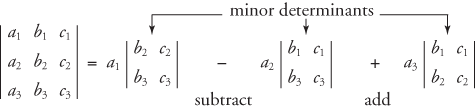Each minor determinant is obtained by crossing out the first column and one row.##### Example 1

Evaluate the following determinant.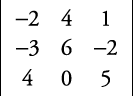First find the minor determinants.The solution is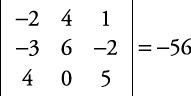To use determinants to solve a system of three equations with three variables (Cramer's Rule), say x, y, and z, four determinants must be formed following this procedure:

1. Write all equations in standard form.

2. Create the denominator determinant, D, by using the coefficients of x, y, and z from the equations and evaluate it.

3. Create the x‐numerator determinant, D x , the y‐numerator determinant, D y , and the z‐numerator determinant, D z , by replacing the respective x, y, and z coefficients with the constants from the equations in standard form and evaluate each determinant.

The answers for x, y, and z are as follows: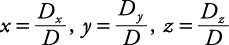##### Example 2

Solve this system of equations, using Cramer's Rule.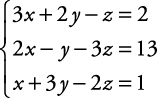Find the minor determinants.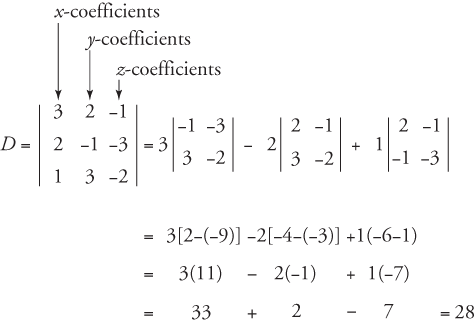Use the constants to replace the x‐coefficients.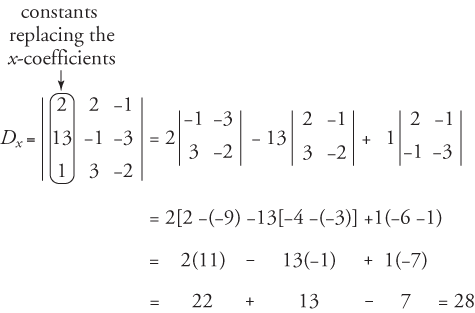Use the constants to replace the y‐coefficients.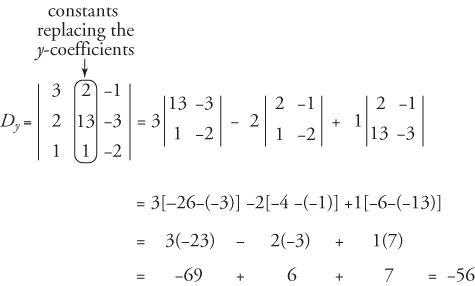Use the constants to replace the z‐coefficients.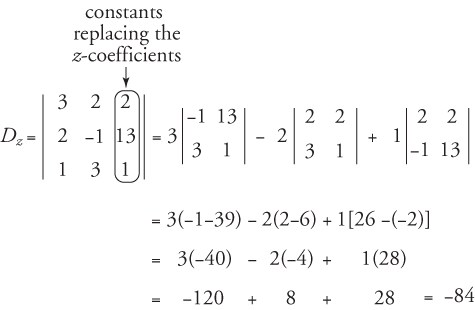Therefore,The check is left to you. The solution is x = 1, y = –2, z = –3.

If the denominator determinant, D, has a value of zero, then the system is either inconsistent or dependent. The system is dependent if all the determinants have a value of zero. The system is inconsistent if at least one of the determinants, D x , D y , or D z , has a value not equal to zero and the denominator determinant has a value of zero.

Back to Top
A18ACD436D5A3997E3DA2573E3FD792A